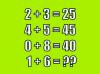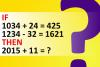BRAIN TEASERSBrain Teasers User Profile

# fatimah aram

rank
603
points
2
See full ranking list
short ranking list
 601 alireza hasibo 2 602 Sheden Mohammed 2 603 fatimah aram 2 604 Tony Midas 2 605 Adel Tarek 2
 Mathematical Puzzle: IF 2+3=... Mathematical Puzzle: IF 2+3=25, 4+5=45 and 0+8=40 THEN 1+6=?Calculate 2015+11 IF 1034+24=425 and 1234-32=1621 THEN 2015+11=?